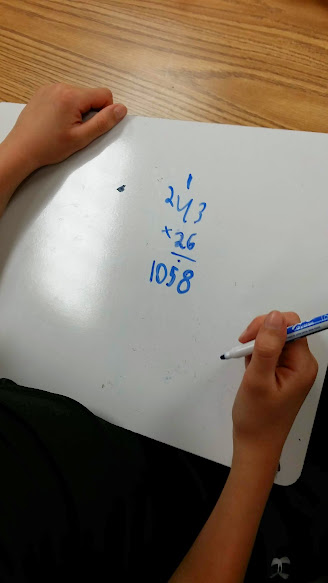# Decimals on a Number Line

13 teachers like this lesson
Print Lesson

## Objective

SWBAT use decimal notation for fractions with denominators 10 or 100 and rewrite 0.62 as 62/100 while placing decimals on a number line.

#### Big Idea

In this lesson students use an inquiry approach to place decimals on a number line. This lesson requires students to use base ten blocks as decimal models in order to build conceptual understanding.

## Warm Up

5 minutes

For this warm up, I ask students to solve a double digit by double digit  multiplication problem on their whiteboards to review 4.NBT.5.

Whiteboards engage the entire class and provides evidence of student learning. Small, personal-sized white boards are inexpensive and if each student has them at their desk they can provide answers and information by simply raising them. Teachers can quickly grasp student understanding and adjust how they move forward.

You can see in this photo students solved 43 x 26.  Note that in this video the student is using the standard traditional algorithm.  It is important to note that I did not teach this standard algorithm shortcut this year, but many of my students eventually discover it on their own by years end.## Concept Development

45 minutes

To make the link between fractions and decimals, students need to understand how the base-ten system can be extended to include numbers less than 1. Fractions that have denominators of 10, 100, and 1,000 and so on are commonly referred to as decimal or base-ten fractions. Focusing on these fractions during early decimal concept development can make the transition between fractions and decimals easier.

This may be many students’ first formal experience working with decimal fractions. This scaffolding task is meant to reinforce fraction comparison skills using visual models, as well as help students make the fractions to decimals connection by using familiar fraction concepts and models to explore numbers that are easily represented by decimals.

I begin this lesson by asking students to model various fractions (denominators of 10 or 100) with base ten blocks.  As students model the fraction, I write the fraction and decimal equivalent on the board.

Next, I tell students that it is possible to convert decimals to fractions by using a number line. and dividing a number line into ten equal parts between each whole number.  I model several examples for students as well as lead a discussion about where numbers like .63 would fall between 0 and 1.

Next, I list several decimals on the board and ask students to place the decimals on their number line. I give each students a large piece of receipt paper so they can make large number lines to work with. I list these numbers: .6, .2, .19, .8, .6 and .9  Students also list the fraction under the number as well as decimals.

In this video you can see a student has a very solid understanding of why .19 is smaller than .2. Notice the model the student is also using.

Notice in this video the student is somewhat confused as to why he would place .19 before .2 and is attempting to explain why. I end up asking this student to model both numbers with base ten blocks in order to help him explain why .19 is smaller.